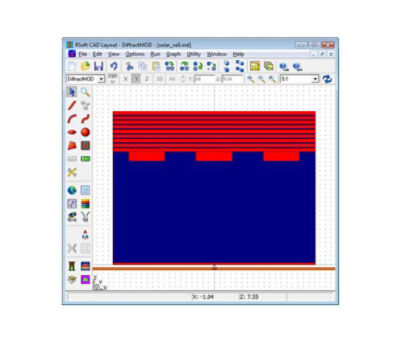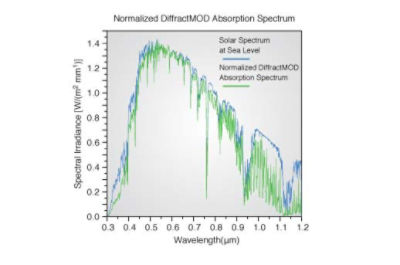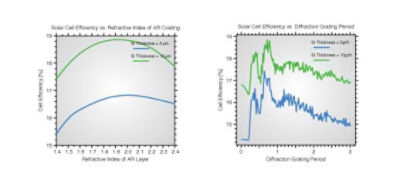# Solar Cell Efficiency

## Tools Used: DiffractMOD, MOST

This example uses DiffractMOD and MOST to determine if the addition of diffractive reflection grating and Distributed Bragg Reflector (DBR) to the design as described in Ref  effectively traps light within the structure resulting in a significant efficiency improvement.

## Structure Overview

The solar cell studied here consists of a simple single-layer AR coating, a silicon absorbing region with frequency-dependent absorption , a silicon rectangular diffraction grating, and a DBR reflector composed of Si/SiO2 layers. This appears in the RSoft CAD as:## Calculating the Absorption Spectrum

While thin-film devices can usually be designed via simple analytical formulas, ray-based optics cannot be used to model diffractive gratings, and a more rigorous approach is required. A simulation tool such as RSoft’s DiffractMOD, which is based on Rigorous Coupled Wave Analysis (RCWA), provides a full-vector solution to model the electro-magnetic fields within the structure and to compute the absorption spectrum of the device. Given this spectrum and the incident solar spectrum (AM 1.5), the total number of photons absorbed can be computed as described in .

An example spectrum, normalized to the solar spectrum, is:## Using MOST to Compute Efficiency

RSoft's scanning and optimization tool, MOST, was configured using a custom post-processing script to automatically compute this efficiency from the absorption spectrum found by DiffractMOD, and then vary selected structural parameters to produce an optimized design. First, the solar cell efficiency was computed without the grating and DBR, and efficiencies of approximately 12% and 15% were found for silicon thicknesses of 5 and 10 μm respectively. Other simulation results were then computed, and are shown below.These results, which show the cell efficiency for different refractive indices of the AR coating and for different grating periods (both with the DBR) show increased efficiencies of approximately 17% and 19%.

## References

 1N. N. Feng, J. Michel, L. Zeng, J. Liu, C. Hong, L. C. Kimerling, and X. Duan, "Design of highly efficient light-trapping structure for thin-film crystalline silicon solar cells," IEEE Trans. on Electron Devices, Vol. 54, No. 8, August, 2007.
 E. D. Palik, Handbook of optical constants of solids, Academic Press, 1985.